# Principles of Video Compression

## Overview

The statistical analysis of video signals indicates that there is a strong correlation both between successive picture frames and within the picture elements themselves. Theoretically, decorrelation of these signals can lead to bandwidth compression without significantly affecting image resolution. Moreover, the insensitivity of the human visual system to loss of certain spatio-temporal visual information can be exploited for further reduction. Hence, subjectively lossy compression techniques can be used to reduce video bit rates while maintaining an acceptable image quality.

For coding still images, only the spatial correlation is exploited. Such a coding technique is called intraframe coding and is the basis for JPEG coding. If temporal correlation is exploited as well, then it is called interframe coding. Interframe predictive coding is the main coding principle that is used in all standard video codecs, such as H.261, H.263, MPEG-1, 2 and 4. It is based on three fundamental redundancy reduction principles:

1. Spatial redundancy reduction: to reduce spatial redundancy among the pixels within a picture (similarity of pixels, within the frames), by employing some data compressors, such as transform coding.
2. Temporal redundancy reduction: to remove similarities between the successive pictures, by coding their differences.
3. Entropy coding: to reduce the redundancy between the compressed data symbols, using variable length coding techniques.

A detailed description of these redundancy reduction techniques is given in the following sections.

## Spatial redundancy reduction

### Predictive coding

In the early days of image compression, both signal processing tools and storage devices were scarce resources. At the time, a simple method for redundancy reduction was to predict the value of pixels based on the values previously coded, and code the prediction error. This method is called differential pulse code modulation (DPCM). Figure 3.1 shows a block diagram of a DPCM codec, where the differences between the incoming pixels from the predictions in the predictor are quantised and coded for transmission. At the decoder the received error signal is added to the prediction to reconstruct the signal. If the quantiser is not used it is called lossless coding, and the compression relies on the entropy coder, which will be explained later.Figure 3.1: Block diagram of a DPCM codec

Best predictions are those from the neighbouring pixels, either from the same frame or pixels from the previous frame, or their combinations. The former is called intraframe predictive coding and the latter is interframe predictive coding. Their combination is called hybrid predictive coding.

It should be noted that, no matter what prediction method is used, every pixel is predictively coded. The minimum number of bits that can be assigned to each prediction error is one bit. Hence, this type of coding is not suitable for low bit rate video coding. Lower bit rates can be achieved if a group of pixels are coded together, such that the average bit per pixel can be less than one bit. Block transform coding is most suitable for this purpose, but despite this DPCM is still used in video compression. For example, interframe DPCM has lower coding latency than interframe block coding. Also, DPCM might be used in coding of motion vectors, or block addresses. If motion vectors in a moving object move in the same direction, coding of their differences will reduce the motion vector information. Of course, the coding would be lossless.

### Transform coding

Transform domain coding is mainly used to remove the spatial redundancies in images by mapping the pixels into a transform domain prior to data reduction. The strength of transform coding in achieving data compression is that the image energy of most natural scenes is mainly concentrated in the low frequency region, and hence into a few transform coefficients. These coefficients can then be quantised with the aim of discarding insignificant coefficients, without significantly affecting the reconstructed image quality. This quantisation process is, however, lossy in that the original values cannot be retained.

To see how transform coding can lead to data compression, consider joint occurrences of two pixels as shown in Figure 3.2.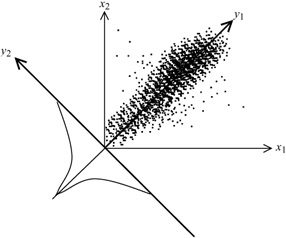Figure 3.2: Joint occurrences of a pair of pixels

Although each pixel x1 or x2 may take any value uniformly between 0 (black) to its maximum value 255 (white), since there is a high correlation (similarity) between them, then it is most likely that their joint occurrences lie mainly on a 45 degrees line, as shown in the Figure. Now if we rotate the x1x2 coordinates by 45 degrees, to a new position y1y2, then the joint occurrences on the new coordinates have a uniform distribution along the y1 axes, but are highly peaked around zero on the y2 axes. Certainly, the bits required to represent the new parameter y1 can be as large as any of x1 or x2, but that of the other parameter y2 is much less. Hence, on average, y1 and y2 can be represented at a lower bit rate than x1 and x2.

Rotation of x1x2 coordinates by 45 degrees is a transformation of vector [x1, x2] by a transformation matrix T:

 (3.1)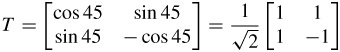Thus, in this example the transform coefficients [y1, y2] become:or

 (3.2)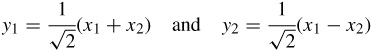y1 is called the average or DC value of x1 and x2 and y2 represents their residual differences. The normalisation factor of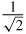makes sure that the signal energy due to transformation is not changed (Parseval theorem). This means that the signal energy in the pixel domain,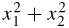, is equal to the signal energy in the transform domain,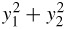. Hence the transformation matrix is orthonormal.

Now, if instead of two pixels we take N correlated pixels, then by transforming the coordinates such that y1 lies on the main diagonal of the sphere, then only the y1 coefficient becomes significant, and the remaining N - 1 coefficients, y2, y3,...,yN, only carry the residual information. Thus, compared with the two pixels case, larger dimensions of transformation can lead to higher compression. Exactly how large the dimensions should be depends on how far pixels can still be correlated to each other. Also, the elements of the transformation matrix, called the basis vectors, have an important role on the compression efficiency. They should be such that only one of the transform coefficients, or at most a few of them, becomes significant and the remaining ones are small.

An ideal choice for the transformation matrix is the one that completely decorrelates the transform coefficients. Thus, if Rxx is the covariance matrix of the input source (pixels), x, then the elements of the transformation matrix T are calculated such that the covariance of the coefficients Ryy = TRxxTT is a diagonal matrix (zero off diagonal elements). A transform that is derived on this basis is the well known Karhunen-Loève transform (KLT) . However, although this transform is optimum, and hence it can give the maximum compression efficiency, it is not suitable for image compression. This is because, as the image statistics change, the elements of the transform need to be recalculated. Thus, in addition to extra computational complexity, these elements need to be transmitted to the decoder. The extra overhead involved in the transmission significantly restricts the overall compression efficiency. Despite this, the KLT is still useful and can be used as a benchmark for evaluating the compression efficiency of other transforms.

A better choice for the transformation matrix is that of the discrete cosine transform (DCT). The reason for this is that it has well defined (fixed) and smoothly varying basis vectors which resemble the intensity variations of most natural images, such that image energy is matched to a few coefficients. For this reason its rate distortion performance closely follows that of the Karhunen-Loève transform, and results in almost identical compression gain . Equally important is the availability of efficient fast DCT transformation algorithms that can be used, especially, in software-based image coding applications .

Since in natural image sequences pixels are correlated in the horizontal and vertical directions as well as in the temporal direction of the image sequence, a natural choice for the DCT is a three-dimensional one. However, any transformation in the temporal domain requires storage of several picture frames, introducing a long delay, which restricts application of transform coding in telecommunications. Hence transformation is confined to two dimensions.

A two-dimensional DCT is a separable process that is implemented using two one-dimensional DCTs: one in the horizontal direction followed by one in the vertical. For a block of M × N pixels, the forward one-dimensional transform of N pixels is given by:

 (3.3)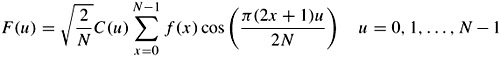where

C(u) =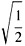for u = 0

C(u) = 1 otherwise

f(x) represents the intensity of the xth pixel, and F(u) represents the N one-dimensional transform coefficients. The inverse one-dimensional transform is thus defined as:

 (3.4)Note that the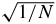normalisation factor is used to make the transformation ortho-normal. That is, the energy in both pixel and transform domains is to be equal. In the standard codecs the normalisation factor for the two-dimensional DCT is defined as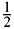. This gives DCT coefficients in the range of -2047 to +2047. The normalisation factor in the pixel domain is then adjusted accordingly (e.g. it becomes 2/N).

To derive the final two-dimensional transform coefficients, N sets of one-dimensional transforms of length M are taken over the one-dimensional transform coefficients of similar frequency in the vertical direction:

 (3.5)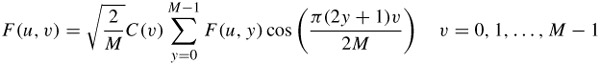where C(v) is defined similarly to C(u).

Thus a block of MN pixels is transformed into MN coefficients. The F(0, 0) coefficient represents the DC value of the block. Coefficient F(0, 1), which is the DC value of all the first one-dimensional AC coefficients, represents the first AC coefficient in the horizontal direction of the block. Similarly, F(1, 0), which is the first AC coefficient of all one-dimensional DC values, represents the first AC coefficient in the vertical direction, and so on.

In practice M = N = 8, such that a two-dimensional transform of 8 x 8 = 64 pixels results in 64 transform coefficients. The choice of such a block size is a compromise between the compression efficiency and the blocking artefacts of coarsely quantised coefficients. Although larger block sizes have good compression efficiency, the blocking artefacts are subjectively very annoying. At the early stage of standardisation of video codecs, the block sizes were made optional at 4 × 4, 8 × 8 and 16 × 16. Now the block size in the standard codecs is 8 × 8.

### Mismatch control

Implementation of both forward and inverse transforms (e.g. eqns 3.3 and 3.4) requires that the cos elements be approximated with finite numbers. Due to this approximation the reconstructed signal, even without any quantisation, cannot be an exact replica of the input signal to the forward transform. For image and video coding applications this mismatch needs to be controlled, otherwise the accumulated error due to approximation can grow out of control resulting in an annoying picture artefact.

One way of preventing error accumulation is to let the error oscillate between two small levels. This guarantees that the accumulated error never exceeds its limit. The approach taken in the standard codecs is to say (e.g. MPEG-2), at the decoder, that the sum of all the values of the 8 × 8 = 64 transform coefficients should be an odd number (no matter whether they are quantised or not). In case the sum is an even number, the value of the highest frequency coefficient, F(7, 7), is either incremented or decremented by 1, depending whether its value itself is odd or even, respectively. This, of course, introduces a very small error, but it cannot be noticed on images for two reasons. First, at the inverse transform, the reconstructed pixels are divided by a large value of the order of N2. Second, since error is introduced by the highest frequency coefficient, it appears as a very high frequency, small amplitude dither-like noise, which is not perceivable at all (the human eye is very tolerant to high frequency noise).

### Fast DCT transform

To calculate transform coefficients, every one-dimensional forward or inverse transformation requires eight multiplications and seven additions. This process is repeated for 64 coefficients, both in the horizontal and vertical directions. Since software-based video compression is highly desirable, methods of reducing such a huge computational burden are also highly desirable.

The fact that DCT is a type of discrete Fourier transform, with the advantage of all-real coefficients, means that one can use a fast transform, similar to the fast Fourier transform, to calculate transform coefficients with complexity proportional to N log2 N, rather than N2. Figure 3.3 shows a butterfly representation of the fast DCT . Intermediate nodes share some of the computational burden, hence reducing the overall complexity. In the Figure, p-p are the inputs to the forward DCT and b-b are the transform coefficients. The inputs can be either the eight pixels for the source image, or eight transform coefficients of the first stage of the one-dimensional transform. Similarly, for inverse transformation, b-b are the inputs to the IDCT, and p-p are the outputs. A C language programme for fast forward DCT is given in Appendix A. In this program, some modifications to the butterfly matrices are made to trade-off the number of additions for multiplications, since multiplications are more computationally intensive than additions. A similar program can be written for the inverse transform.Figure 3.3: A fast DCT flow chart

## Quantisation of DCT coefficients

The domain transformation of the pixels does not actually yield any compression. A block of 64 pixels is transformed into 64 coefficients. Due to the orthonormality of the transformation, the energy in both the pixel and the transform domains are equal, hence no compression is achieved. However, transformation causes the significant part of the image energy to be concentrated at the lower frequency components, with the majority of the coefficients having little energy. It is the quantisation and variable length coding of the DCT coefficients that lead to bit rate reduction. Moreover, by exploiting the human eye's characteristics, which are less sensitive to picture distortions at higher frequencies, one can apply even coarser quantisation at these frequencies, to give greater compression. Coarser quantisation step sizes force more coefficients to zero and as a result more compression is gained, but of course the picture quality deteriorates accordingly.

The class of quantiser that has been used in all standard video codecs is based around the so-called uniform threshold quantiser (UTQ). It has equal step sizes with reconstruction values pegged to the centriod of the steps. This is illustrated in Figure 3.4.Figure 3.4: Quantisation characteristics

The two key parameters that define a UTQ are the threshold value, th, and the step size, q. The centroid value is typically defined mid way between quantisation intervals. Note that, although AC transform coefficients have nonuniform characteristics, and hence can be better quantised with nonuniform quantiser step sizes (the DC coefficient has a fairly uniform distribution), bit rate control would be easier if they were quantised linearly. Hence, a key property of UTQ is that the step sizes can be easily adapted to facilitate rate control.

A further two subclasses of UTQ can be identified within the standard codecs, namely those with and without a dead zone. These are illustrated in Figure 3.5 and will be hereafter abbreviated as UTQ-DZ and UTQ, respectively. The term dead zone commonly refers to the central region of the quantiser, whereby the coefficients are quantised to zero.Figure 3.5: Uniform quantisers (a with dead zone) (b without dead zone)

Typically, UTQ is used for quantising intraframe DC, F(0, 0), coefficients, and UTQ-DZ is used for the AC and the DC coefficients of interframe prediction error. This is intended primarily to cause more nonsignificant AC coefficients to become zero, so increasing the compression. Both quantisers are derived from the generic quantiser of Figure 3.4, where in UTQ th is set to zero but in UTQ-DZ it is set to q/2 and in the most inner region it is allowed to vary between q/2 and q just to increase the number of zero-valued outputs, as shown in Figure 3.5. Thus the dead zone length can be from q to 2q. In some implementations (e.g. H.263 or MPEG-4), the decision and/or the reconstruction levels of the UTQ-DZ quantiser might be shifted by q/4 or q/2.

In practice, rather than transmitting a quantised coefficient to the decoder, its ratio to the quantiser step size, called the quantisation index, I

 (3.6)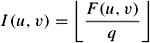is transmitted. (In eqn. 3.6 the symbol ⌊.⌋ stands for rounding to the nearest integer.) The reason for defining the quantisation index is that it has a much smaller entropy than the quantised coefficient. At the decoder, the reconstructed coefficients, Fq(u, v), after inverse quantisation are given by:

 (3.7)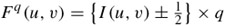If required, depending on the polarity of the index, an addition or subtraction of half the quantisation step is required to deliver the centroid representation, reflecting the quantisation characteristics of Figure 3.5.

It is worth noting that, for the standard codecs, the quantiser step size q is fixed at 8 for UTQ but varies from 2–62, in even step sizes, for the UTQ-DZ. Hence the entire quantiser range, or the quantiser parameter Qp (half the quantiser step size), can be defined with five bits (1–31).

Uniform quantisers with and without a dead zone can also be used in DPCM coding of pixels (section 3.1). Here, the threshold is set to zero, th = 0, and the quantisers are usually identified with even and odd numbers of levels, respectively.

One of the main problems of linear quantisers in DPCM is that for lower bit rates the number of quantisation levels is limited and hence the quantiser step size is large. In coding of plain areas of the picture, if a quantiser with an even number of levels is used, then the reconstructed pixels oscillate between -q/2 and q/2. This type of noise at these areas, in particular at low luminance levels, is visible and is called granular noise.

Larger quantiser step sizes with an odd number of levels (dead zone) reduce the granular noise, but cause loss of pixel resolution at the plain areas. This type of noise when the quantiser step size is relatively large is annoying and is called contouring noise.

To reduce granular and contouring noises, the quantiser step size should be reduced. This of course for a limited number of quantisation levels (low bit rate) reduces the outmost reconstruction level. In this case large pixel transitions such as sharp edges cannot be coded with good fidelity. It might take several cycles for the encoder to code one large sharp edge. Hence, edges appear smeared and this type of noise is known as slope overload noise.

In order to reduce the slope overload noise without increasing the granular or contouring noise, the quantiser step size can change adaptively. For example, a lower step size quantiser is used at the plain areas and a larger step size is employed at the edges and high texture areas. Note that the overhead of adaptation can be very costly (e.g. one bit per pixel).

The other method is to use a nonlinear quantiser with small step sizes at the inner levels and larger step sizes at the outer levels. This suits DPCM video better than the linear quantiser. Nonlinear quantisers reduce the entropy of the data more than linear quantisers. Hence data is less dependent on the variable length codes (VLC), increasing the robustness of the DPCM video to channel errors.

## Temporal redundancy reduction

By using the differences between successive images, temporal redundancy is reduced. This is called interframe coding. For static parts of the image sequence, temporal differences will be close to zero, and hence are not coded. Those parts that change between the frames, either due to illumination variation or to motion of the objects, result in significant image error, which needs to be coded. Image changes due to motion can be significantly reduced if the motion of the object can be estimated, and the difference is taken on the motion compensated image.

Figure 3.6 shows the interframe error between successive frames of the Claire test image sequence and its motion compensated counterpart. It is clear that motion compensation can substantially reduce the interframe error.Figure 3.6: (a Interframe) (b motion compensated interframe)

### Motion estimation

To carry out motion compensation, the motion of the moving objects has to be estimated first. This is called motion estimation. The commonly used motion estimation technique in all the standard video codecs is the block matching algorithm (BMA). In a typical BMA, a frame is divided into blocks of M × N pixels or, more usually, square blocks of N2 pixels . Then, for a maximum motion displacement of w pixels per frame, the current block of pixels is matched against a corresponding block at the same coordinates but in the previous frame, within the square window of width N + 2w (Figure 3.7). The best match on the basis of a matching criterion yields the displacement.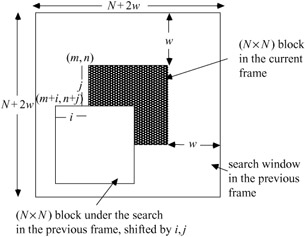Figure 3.7: The current and previous frames in a search window

Various measures such as the cross correlation function (CCF), mean-squared error (MSE) and mean absolute error (MAE) can be used in the matching criterion [4–6]. For the best match, in the CCF the correlation has to be maximised, whereas in the latter two measures the distortion must be minimised. In practical coders both MSE and MAE are used, since it is believed that CCF would not give good motion tracking, especially when the displacement is not large . The matching functions of the type MSE and MAE are defined as, for MSE: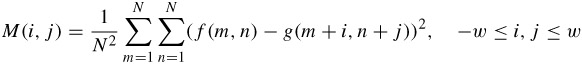and for MAE:where f(m, n) represents the current block of N2 pixels at coordinates (m, n) and g(m + i, n + j) represents the corresponding block in the previous frame at new coordinates (m + i, n + j). At the best matched position of i = a and j = b, the motion vector MV(a, b), represents the displacement of all the pixels within the block.

To locate the best match by full search, (2w + 1)2 evaluations of the matching criterion are required. To reduce processing cost, MAE is preferred to MSE and hence is used in all the video codecs. However, for each block of N2 pixels we still need to carry out (2w + 1)2 tests, each with almost 2N2 additions and subtractions. This is still far from being suitable for the implementation of BMA in software-based codecs. Measurements of the video encoders' complexity show that motion estimations comprise almost 50–70 per cent of the overall encoder's complexity . This of course depends on the motion activity in the scene and whether a fast DCT is used in deriving the transform coefficients. For example, the percentage of the processing time required to calculate the motion vectors of the Mobile and Claire test image sequences in an MPEG-1 software-based encoder is given in Table 3.1. Note that although more processing time is required for motion estimation in B-pictures than P-pictures, since the search ranges in P-pictures are much larger than for B-pictures, the overall processing time for motion estimation in P-pictures can be larger than that of the B-pictures, as shown in the Table. The reason for these will be dealt with in Chapter 7, when we talk about different picture types in the MPEG-1 encoder. In any case, as motion estimation is a costly process, fast motion estimation techniques are highly desirable.

Table 3.1: Percentage of processing time required to carry out motion estimation in an MPEG-1 encoder

Category

Fast DCT

Brute force DCT

Picture type

Mobile

Claire

Mobile

Claire

P-frame ME

66.1 %

68.4%

53.3%

56.1 %

B -frame ME

58.2%

60.9%

46.2%

48.7%

### Fast motion estimation

In the past two decades a number of fast search methods for motion estimation have been introduced to reduce the computational complexity of BMA. The basic principle of these methods is that the number of search points can be reduced, by selectively checking only a small number of specific points, assuming that the distortion measure monotonically decreases towards the best matched point. Jain and Jain  were the first to use a two-dimensional logarithmic (TDL) search method to track the direction of a minimum mean-squared error distortion measure. In their method, the distortion for the five initial positions, one at the centre of the coordinate and four at coordinates (±w/2, ±w/2) of the search window, are computed first. In the next step, three more positions with the same step size in the direction of the previous minimum position are searched. The step size is then halved and the above procedure is continued until the step size becomes unity. Finally, all the nine positions are searched. With this method, for w = 5 pixels/frame, 21 positions are searched as opposed to 121 positions required in the full search method.

Koga et al.  use a three-step search (TSS) method to compute motion displacements up to six pixels/frame. In their method all eight positions surrounding the coordinate with a step size of w/2 are searched first. At each minimum position the search step size is halved and the next eight new positions are searched. This method, for w = 6 pixels/frame, searches 25 positions to locate the best match. The technique is the recommended method for the test of software-based H.261  for videophone applications.

In Kappagantula and Rao's  modified motion estimation algorithm (MMEA), prior to halving the step sizes, two more positions are also searched. With this method for w = 7 pixels/frame, only 19 MAE computations are required. In Srinivasan and Rao's  conjugate direction search (CDS) method, at every iteration of the direction search two conjugate directions with a step size of one pixel, centred at the minimum position, are searched. Thus, for w = 5 pixels/frame, there will be only 13 searches at most.

Another method of fast BMA is the cross search algorithm (CSA) . In this method, the basic idea is still a logarithmic step search, which has also been exploited in [4,6,8], but with some differences, which lead to fewer computational search points. The main difference is that at each iteration there are four search locations which are the end points of a cross (×) rather than (+). Also, at the final stage, the search points can be either the end points of (×) or (+) crosses, as shown in Figure 3.8. For a maximum motion displacement of w pixels/frame, the total number of computations becomes 5 + 4 log2 w.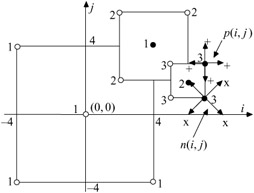Figure 3.8: An example of the CSA search for w = 8 pixels/frame

Puri et al.  have introduced the orthogonal search algorithm (OSA) in which, with a logarithmic step size, at each iteration four new locations are searched. This is the fastest method of all known fast MBAs. In this method, at every step, two positions are searched alternately in the vertical and horizontal directions. The total number of test points is 1 + 4 log2 w.

Table 3.2 shows the computational complexity of various fast search methods, for a range of motion speeds from 4 to 16 pixels/frame. The motion compensation efficiency of these algorithms for a motion speed of w = 8 pixels/frame for two test image sequences is tabulated in Table 3.3.

Table 3.2: Computational complexity

Algorithm

Maximum number of search points

w

4

8

16

FSM

(2w + 1)2

81

289

1089

TDL

2 + 7 log2 w

16

23

30

TSS

1 + 8 log2 w

17

25

33

MMEA

1 + 6 log2 w

13

19

25

CDS

3 + 2w

11

19

35

OSA

1 + 4 log2 w

9

13

17

CSA

5 + 4 log2 w

13

17

21

Table 3.3: Compensation efficiency

Algorithm

Split screen

Trevor white

entropy (bits/pel)

standard deviation

entropy (bits/pel)

standard deviation

FSM

4.57

7.39

4.41

6.07

TDL

4.74

8.23

4.60

6.92

TSS

4.74

8.19

4.58

6.86

MMEA

4.81

8.56

4.69

7.46

CDS

4.84

8.86

4.74

7.54

OSA

4.85

8.81

4.72

7.51

CSA

4.82

8.65

4.68

7.42

It can be seen that, although fast search methods reduce the computational complexity of the full search method (FSM) significantly, their motion estimation accuracy (compensation efficiency) has not been degraded noticeably.

### Hierarchical motion estimation

The assumption of monotonic variation of image intensity employed in the fast BMAs often causes false estimations, especially for larger picture displacements. These methods perform well for slow moving objects, such as those in video conferencing. However, for higher motion speeds, due to the intrinsic selective nature of these methods, they often converge to a local minimum of distortion.

One method of alleviating this problem is to subsample the image to smaller sizes, such that the motion speed is reduced by the sampling ratio. The process is done on a multilevel image pyramid, known as the hierarchical block matching algorithm (HBMA) . In this technique, pyramids of the image frames are reconstructed by successive two-dimensional filtering and subsampling of the current and past image frames. Figure 3.9 shows a three-level pyramid, where for simplicity each level of the upper level of the pyramid is taken as the average of four adjacent pixels of one level below. Effectively this is a form of lowpass filtering.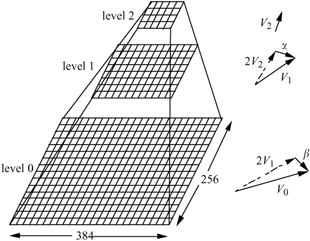Figure 3.9: A three-level image pyramid

Conventional block matching, either full search or any fast method, is first applied to the highest level of the pyramid (level 2 in Figure 3.9). This motion vector is then doubled in size, and further refinement within one pixel search is carried out in the following level. The process is repeated to the lowest level. Therefore, with an n-level pyramid the maximum motion speed of w at the highest level is reduced to w/2n-1.

For example, a maximum motion speed of 32 pixels/frame with a three-level pyramid is reduced to eight pixels/frame, which is quite manageable by any fast search method. Note also that this method can be regarded as another type of fast search with a performance very close to the full search irrespective of the motion speed, but the computational complexity can be very close to the fast logarithmic methods.

As an example, for a maximum motion speed of 32 pixels/frame, which is very common in high definition video or most TV sports programmes (particularly in the P-pictures of the standard codecs, which can be several frames apart from each other) although the nonhierarchical full search BMA requires (2 × 32 + 1)2 = 4225 operations, a four-level hierarchy, where the motion speed at the top level is 32/24-1 = 4 pixels/frame, only requires (2 × 4 + 1)2 + 3 × 9 = 108 operations. Here with the full search method 81 operations are carried out at the top level, and at each lower level nine new positions are searched.

## Variable length coding

For further bit rate reduction, the transform coefficients and the coordinates of the motion vectors are variable length coded (VLC). In VLC, short code words are assigned to the highly probable values and long code words to the less probable ones. The lengths of the codes should vary inversely with the probability of occurrences of the various symbols in VLC. The bit rate required to code these symbols is the inverse of the logarithm of probability, p, at base 2 (bits), i.e. log2 p. Hence, the entropy of the symbols which is the minimum average bits required to code the symbols can be calculated as:

 (3.9)There are two types of VLC, which are employed in the standard video codecs. They are Huffman coding and arithmetic coding. It is noted that Huffman coding is a simple VLC code, but its compression can never reach as low as the entropy due to the constraint that the assigned symbols must have an integral number of bits. However, arithmetic coding can approach the entropy since the symbols are not coded individually . Huffman coding is employed in all standard codecs to encode the quantised DCT coefficients as well as motion vectors. Arithmetic coding is used, for example, in JPEG, JPEG2000, H.263 and shape and still image coding of MPEG-4 [15–17], where extra compression is demanded.

### Huffman coding

Huffman coding is the most commonly known variable length coding method based on probability statistics. Huffman coding assigns an output code to each symbol with the output codes being as short as 1 bit, or considerably longer than the input symbols, depending on their probability. The optimal number of bits to be used for each symbol is - log2 p, where p is the probability of a given symbol.

However, since the assigned code words have to consist of an integral number of bits, this makes Huffman coding suboptimum. For example, if the probability of a symbol is 0.33, the optimum number of bits to code that symbol is around 1.6 bits, but the Huffman coding scheme has to assign either one or two bits to the code. In either case, on average it will lead to more bits compared to its entropy. As the probability of a symbol becomes very high, Huffman coding becomes very nonoptimal. For example, for a symbol with a probability of 0.9, the optimal code size should be 0.15 bits, but Huffman coding assigns a minimum value of one bit code to the symbol, which is six times larger than necessary. Hence, it can be seen that resources are wasted.

To generate the Huffman code for symbols with a known probability of occurrence, the following steps are carried out:

• rank all the symbols in the order of their probability of occurrence
• successively merge every two symbols with the least probability to form a new composite symbol, and rerank order them; this will generate a tree, where each node is the probability of all nodes beneath it
• trace a path to each leaf, noting the direction at each node.

Figure 3.10 shows an example of Huffman coding of seven symbols, A-G. Their probabilities in descending order are shown in the third column. In the next column the two smallest probabilities are added and the combined probability is included in the new order. The procedure continues to the last column, where a single probability of 1 is reached. Starting from the last column, for every branch of probability a 0 is assigned on the top and a 1 in the bottom, shown in bold digits in the Figure. The corresponding codeword (shown in the first column) is read off by following the sequence from right to left. Although with fixed word length each sample is represented by three bits, they are represented in VLC from two to four bits.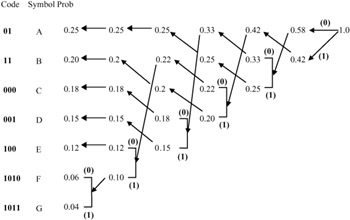Figure 3.10: An example of Huffman code for seven symbols

The average bit per symbol is then:

0.25 × 2 + 0.20×2 + 0.18×3 + 0.15×3 + 0.12×3 + 0.06×4 + 0.04×4 = 2.65 bits

which is very close to the entropy given by:It should be noted that for a large number of symbols, such as the values of DCT coefficients, such a method can lead to a long string of bits for the very rarely occurring values, and is impractical. In such cases normally a group of symbols is represented by their aggregate probabilities and the combined probabilities are Huffman coded, the so-called modified Huffman code. This method is used in JPEG. Another method, which is used in H.261 and MPEG, is two-dimensional Huffman, or three-dimensional Huffman in H.263 [9,16].

### Arithmetic coding

Huffman coding can be optimum if the symbol probability is an integer power of, which is usually not the case. Arithmetic coding is a data compression technique that encodes data by creating a code string, which represents a fractional value on the number line between 0 and 1 . It encourages clear separation between the model for representing data and the encoding of information with respect to that model. Another advantage of arithmetic coding is that it dispenses with the restriction that each symbol must translate into an integral number of bits, thereby coding more efficiently. It actually achieves the theoretical entropy bound to compression efficiency for any source. In other words, arithmetic coding is a practical way of implementing entropy coding.

There are two types of modelling used in arithmetic coding: the fixed model and the adaptive model. Modelling is a way of calculating, in any given context, the distribution of probabilities for the next symbol to be coded. It must be possible for the decoder to produce exactly the same probability distribution in the same context. Note that probabilities in the model are represented as integer frequency counts. Unlike the Huffman-type, arithmetic coding accommodates adaptive models easily and is computationally efficient. The reason why data compression requires adaptive coding is that the input data source may change during encoding, due to motion and texture.

In the fixed model, both encoder and decoder know the assigned probability to each symbol. These probabilities can be determined by measuring frequencies in representative samples to be coded and the symbol frequencies remain fixed. Fixed models are effective when the characteristics of the data source are close to the model and have little fluctuation.

In the adaptive model, the assigned probabilities may change as each symbol is coded, based on the symbol frequencies seen so far. Each symbol is treated as an individual unit and hence there is no need for a representative sample of text. Initially, all the counts might be the same, but they update, as each symbol is seen, to approximate the observed frequencies. The model updates the inherent distribution so the prediction of the next symbol should be close to the real distribution mean, making the path from the symbol to the root shorter.

Due to the important role of arithmetic coding in the advanced video coding techniques, in the following sections a more detailed description of this coding technique is given.

3.4.2.1 Principles of arithmetic coding

The fundamental idea of arithmetic coding is to use a scale in which the coding intervals of real numbers between 0 and 1 are represented. This is in fact the cumulative probability density function of all the symbols which add up to 1. The interval needed to represent the message becomes smaller as the message becomes longer, and the number of bits needed to specify that interval is increased. According to the symbol probabilities generated by the model, the size of the interval is reduced by successive symbols of the message. The more likely symbols reduce the range less than the less likely ones and hence they contribute fewer bits to the message.

To explain how arithmetic coding works, a fixed model arithmetic code is used in the example for easy illustration. Suppose the alphabet is {a, e, i, o, u, !} and the fixed model is used with the probabilities shown in Table 3.4.

Table 3.4: Example: fixed model for alphabet {a, e, i, o, u, !}

Symbol

Probability

Range

a

0.2

[0.0, 0.2)

e

0.3

[0.2, 0.5)

i

0.1

[0.5, 0.6)

o

0.2

[0.6, 0.8)

u

0.1

[0.8, 0.9)

!

0.1

[0.9, 1.0)

Once the symbol probability is known, each individual symbol needs to be assigned a portion of the [0, 1) range that corresponds to its probability of appearance in the cumulative density function. Note also that the character with a range of [lower, upper) owns everything from lower up to, but not including, the upper value. So, the alphabet u with probability 0.1, defined in the cumulative range of [0.8, 0.9), can take any value from 0.8 to 0.8999 ....

The most significant portion of an arithmetic coded message is the first symbol to be encoded. Using an example that a message eaii! is to be coded, the first symbol to be coded is e. Hence, the final coded message has to be a number greater than or equal to 0.2 and less than 0.5. After the first character is encoded, we know that the lower number and the upper number now bound our range for the output. Each new symbol to be encoded will further restrict the possible range of the output number during the rest of the encoding process.

The next character to be encoded, a, is in the range of 0-0.2 in the new interval. It is not the first number to be encoded, so it belongs to the range corresponding to 0-0.2, but in the new subrange of [0.2, 0.5). This means that the number is now restricted to the range of [0.2, 0.26), since the previous range was 0.3 (0.5 - 0.2 = 0.3) units long and one-fifth of that is 0.06. The next symbol to be encoded, i, is in the range of [0.5, 0.6), that corresponds to 0.5-0.6 in the new subrange of [0.2, 0.26) and gives the smaller range [0.23, 0.236). Applying this rule for coding of successive characters, Table 3.5 shows the successive build up of the range of the message coded so far.

Table 3.5: Representation of arithmetic coding process

New character

Range

Initially:

[0, 1)

After seeing a symbol:

e

[0.2, 0.5)

a

[0.2, 0.26)

i

[0.23, 0.236)

i

[0.233, 0.2336)

!

[0.23354, 0.2336)

Figure 3.11 shows another representation of the encoding process. The range is expanded to fill the whole range at every stage and marked with a scale that gives the end points as a number. The final range, [0.23354, 0.2336) represents the message eaii!. This means that if we transmit any number in the range of 0.23354 ≤ x < 0.2336, that number represents the whole message of eaii!.Figure 3.11: Representation of arithmetic coding process with the interval scaled up at each stage for the message eaii!

Given this encoding scheme, it is relatively easy to see how during the decoding the individual elements of the eaii! message are decoded. To verify this, suppose a number x = 0.23355 in the range of 0.23354 ≤ x < 0.2336 is transmitted. The decoder, using the same probability intervals as the encoder, performs a similar procedure. Starting with the initial interval [0, 1), only the interval [0.2, 0.5) of e envelops the transmitted code of 0.23355. So the first symbol can only be e. Similar to the encoding process, the symbol intervals are then defined in the new interval [0.2, 0.5). This is equivalent to defining the code within the initial range [0, 1), but offsetting the code by the lower value and then scaling up within its original range. That is, the new code will be (0.23355 - 0.2)/(0.5 - 0.2) = 0.11185, which is enveloped by the interval [0.0, 0.2) of symbol a. Thus the second decoded symbol is a. To find the third symbol, a new code within the range of a should be found, i.e. (0.11185 - 0.0)/(0.2 - 0.0) = 0.55925. This code is enveloped by the range of [0.5, 0.6) of symbol i and the resulting new code after decoding the third symbol will be (0.55925 - 0.5)/(0.6 - 0.5) = 0.5925, which is again enveloped by [0.5, 0.6). Hence the fourth symbol will be i. Repeating this procedure will yield a new code of (0.5925 - 0.5)/(0.6 - 0.5) = 0.925. This code is enveloped by [0.9, 1), which decodes symbol !, the end of decoding symbol, and the decoding process is terminated. Table 3.6 shows the whole decoding process of the message eaii!.

Table 3.6: Representation of decoding process of arithmetic coding

Encoded number

Output symbol

Range

0.23355

e

[0.2, 0.5)

0.11185

a

[0.0, 0.2)

0.55925

i

[0.5, 0.6)

0.59250

i

[0.5, 0.6)

0.92500

!

[0.9, 1.0)

In general, the decoding process can be formulated as:

 (3.10)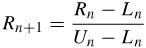where Rn is a code within the range of lower value Ln and upper value Un of the nth symbol and Rn+1 is the code for the next symbol.

3.4.2.2 Binary arithmetic coding

In the preceding section we saw that, as the number of symbols in the message increases, the range of the message becomes smaller. If we continue coding more symbols then the final range may even become smaller than the precision of any computer to define such a range. To resolve this problem we can work with binary arithmetic coding.

In Figure 3.11 we saw that after each stage of coding, if we expand the range to its full range of [0, 1), the apparent range is increased. However, the values of the lower and upper numbers are still small. Therefore, if the initial range of [0, 1) is replaced by a larger range of [0, MAX_VAL), where MAX_VAL is the largest integer number that a computer can handle, then the precision problem is resolved. If we use 16-bit integer numbers, then MAX_VAL= 216 - 1. Hence, rather than defining the cumulative probability in the range of [0,1), we define their cumulative frequencies scaled up within the range of [0, 216 - 1).

At the start, the coding interval [lower, upper) is initialised to the whole scale [0, MAX_VAL). The model's frequencies, representing the probability of each symbol in this range, are also set to their initial values in the range. To encode a new symbol element ek assuming that symbols e1... ek-1 have already being coded, we project the model scale to the interval resulting from the sequence of events. The new interval [lower', upper') for the sequence e1... ek is calculated from the old interval [lower, upper) of the sequence e1 ... ek-1 as follows:

 lower' = lower + width * low/maxfreq width' = width * symb_width/maxfreq upper' = lower' + width' = lower + width * (low + symb_width)/maxfreq = lower + width * up/maxfreq

with:

• width = upper - lower (old interval)
• width' = upper' - lower' (new interval)
• symb_width = up - low (model's frequency)

At this stage of the coding process, we do not need to keep the previous interval [lower, upper) in the memory, so we allocate the new values [lower', upper'). We then compare the new interval with the scale [0, MAX_VAL) to determine whether there is any bit for transmission down the channel. These bits are due to the redundancy in the binary representation of lower and upper values. For example, if values of both lower and upper are less than half the [0, MAX_VAL) range, then their most significant number in binary form is 0. Similarly, if both belong to the upper half range, their most significant number is 1. Hence we can make a general rule:

• if lower and upper levels belong to the first half of the scale, the most significant bit for both will be 0
• if lower and upper belong to the second half of the scale, their most significant bit will be 1
• otherwise, when lower and upper belong to the different halves of the scale their most significant bits will be different (0 and 1).

Thus for the cases where lower and upper values have the same most significant bit, we send this bit down the channel and calculate the new interval as follows:

• sending a 0 corresponds to removal of the second half of the scale and keeping its first half only; the new scale is expanded by a factor 2 to obtain its representation in the whole scale of [0, MAX_VAL) again, as shown in Figure 3.12Figure 3.12: Both lower and upper values in the first half

• sending a 1 corresponds to the shift of the second half of the scale to its first half; that is subtracting half of the scale value and multiplying the result by a factor 2 to obtain its representation in the whole scale again, as shown in Figure 3.13.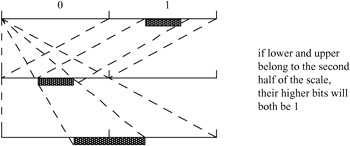Figure 3.13: Both lower and upper values in the second half

If the interval always remains in either half of the scale after a bit has been sent, the operation is repeated as many times as necessary to obtain an interval occupying both halves of the scale. The complete procedure is called the interval testing loop.

Now we go back to the case where both of the lower and upper values are not in either of the half intervals. Here we identify two cases: first the lower value is in the second quarter of the scale and the upper value is in the third quarter. Hence the range of frequency is less than 0.5. In this case, in the binary representation, the two most significant bits are different, 01 for the lower and 10 for the upper, but we can deduce some information from them. That is, in both cases, the second bit is the complementary bit to the first. Hence, if we can find out the second bit, the previous bit is its complement. Therefore, if the second bit is 1, then the previous value should be 0, and we are in the second quarter, meaning the lower value. Similar conclusions can be drawn for the upper value.

Thus we need to divide the interval within the scale of [0, MAX_VAL) into four segments instead of two. Then the scaling and shifting for this case will be done only on the portion of the scale containing second and third quarters, as shown in Figure 3.14.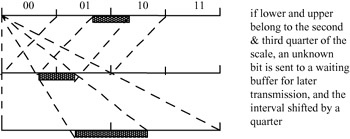Figure 3.14: Lower and upper levels in the second and third quarter, respectively

Thus the general rule for this case is that:

• if the interval belongs to the second and third quarters of the scale: an unknown bit ? is sent to a waiting buffer for later definition and the interval transformed as shown in Figure 3.14
• if the interval belongs to more than two different quarters of the scale (the interval occupies parts of three or four different quarters), there is no need to transform the coding interval; the process exits from this loop and goes to the next step in the execution of the program; this means that if the probability in that interval is greater than 0.5, no bits are transmitted; this is the most important part of arithmetic coding which leads to a low bit rate.

A flow chart of the interval testing loop is shown in Figure 3.15 and a detailed example of coding a message is given in Figure 3.16. For every symbol to be coded we invoke the flow chart of Figure 3.15, except where the symbol is the end of file symbol, where the program stops. After each testing loop, the model can be updated (frequencies modified if the adaptive option has been chosen).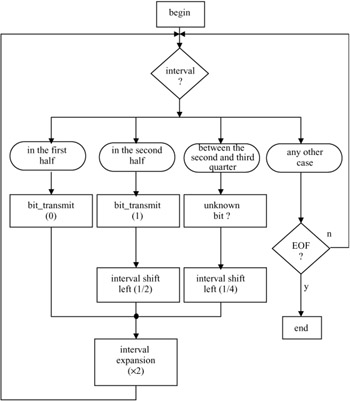Figure 3.15: A flow chart for binary arithmetic coding

```#define q1 16384
#define q2 32768
#define q3 49152
#define top 65535

static long low, high, opposite_bits, length;
void encode_a_symbol (int index, int cumul_freq[])
{
length = high - low +1;
high = low -1 + (length * cumul_freq[index]) / cumul_freq ;
low += (length * cumul_freq[index+1])/ cumul_freq ;
for( ; ; ){
if(high  0) {
send out a bit "1" to PSC_FIFO;
opposite_bits--;
}
}
else if (low >= q2) {
send out a bit "1" to PSC_FIFO;
while (opposite_bits > 0) {
send out a bit "0" to PSC_FIFO;
opposite_bits--;
}
low -= q2;
high -= q2;
}
else if (low >= q1 && high < q3) {
opposite_bits += 1;
low -= q1;
high -= q1;
}
else break;
low *= 2;
high = 2 * high + 1;
}
}
```

Figure 3.16: A C program of binary arithmetic coding

A C program of binary arithmetic coding is given in Figure 3.16.

The values of low and high are initialised to 0 and top, respectively. PSC_FIFO is first-in-first-out (FIFO) for buffering the output bits from the arithmetic encoder. The model is specified through cumul_freq [ ], and the symbol is specified using its index in the model.

3.4.2.3 An example of binary arithmetic coding

Because of the complexity of the arithmetic algorithm, a simple example is given below. To simplify the operations further, we use a fixed model with four symbols a, b, c and d, where their fixed probabilities and cumulative probabilities are given in Table 3.7, and the message sequence of events to be encoded is bbacd.

Table 3.7: Probability and cumulative probability of four symbols as an example

Symbol

pdf

cdf

a

0.3

[0.0, 0.3)

b

0.5

[0.3, 0.8)

c

0.1

[0.8, 0.9)

d

0.1

[0.9, 1.0)

Considering what we saw earlier, we start by initialising the whole range to [0, 1). In sending b, which has a range of [0.3, 0.8), since lower = 0.3 is in the second quarter and upper = 0.8 in the fourth quarter (occupying more than two quarters) nothing is sent, and no scaling is required, as shown in Table 3.8.

Table 3.8: Generated binary bits of coding bbacd message

Encoding

Coding interval

Width

Bit

initialisation:

[0, 1)

1.000

after b:

[0.3, 0.8)

0.500

after bb:

[0.45, 0.7)

0.250

after interval test

[0.4, 0.9)

0.500

?

after bba:

[0.40, 0.55)

0.150

after interval test

[0.3, 0.6)

0.300

?

after interval test

[0.1, 0.7)

0.600

?

after bbac:

[0.58, 0.64)

0.060

after interval test

[0.16, 0.28)

0.120

1000

after interval test

[0.32, 0.56)

0.240

0

after interval test

[0.14, 0.62)

0.480

?

after bbacd:

[0.572, 0.620)

0.048

after interval test

[0.144, 0.240)

0.096

10

after interval test

[0.288, 0.480)

0.192

0

after interval test

[0.576, 0.960)

0.384

0

after interval test

[0.152, 0.920)

0.768

1

To send the next b, i.e. bb, since the previous width = 0.8 - 0.3 = 0.5, the new interval becomes [0.3 + 0.3 x 0.5, 0.3 + 0.8 x 0.5) = [0.45, 0.7). This time lower = 0.45 is in the second quarter but upper = 0.7 is in the third quarter, so the unknown bit ? is sent to the buffer, such that its value is to be determined later.

Note that since the range [0.45, 0.7) covers the second and third quarters, according to Figure 3.14, we have to shift both of them by a quarter (0.25) and then magnify by a factor of 2, i.e. the new interval is [(0.45 - 0.25) x 2, (0.7 - 0.25) x 2) = [0.4, 0.9) which has a width of 0.5. To code the next symbol a, the range becomes [0.4 + 0 x 0.5, 0.4 + 0.3 × 0.5) = [0.4, 0.55). Again since lower and upper are at the second and third quarters, the unknown ? is stored. According to Figure 3.14 both quarters are shifted by 0.25 and magnified by 2, [(0.4 - 0.25) × 2, (0.55 - 0.25) × 2) = [0.3, 0.6).

Again [0.3, 0.6) is in the second and third interval so another ? is stored. If we shift and magnify again [(0.3 - 0.25) × 2, (0.6 - 0.25) × 2) = [0.1, 0.7), which now lies in the first and third quarters, so nothing is sent if there is no scaling. Now if we code c, i.e. bbac, the interval becomes [0.1 + 0.8 × 0.6, 0.1 + 0.9 × 0.6) = [0.58, 0.64).

Now since [0.58, 0.64) is in the second half we send 1. We now go back and convert all ? to 000 complementary to 1. Thus we have sent 1000 so far. Note that bits belonging to ? are transmitted after finding a 1 or 0. Similarly, the subsequent symbols are coded and the final generated bit sequence becomes 1000010001. Table 3.8 shows this example in a tabular representation. A graphical representation is given in Figure 3.17.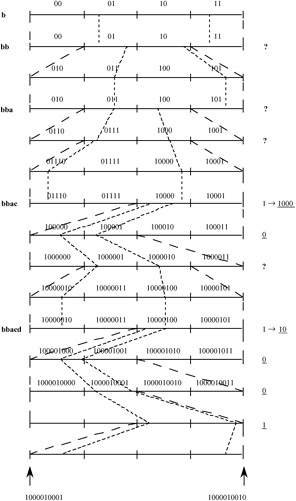Figure 3.17: Derivation of the binary bits for the given example

3.4.2.4 Adaptive arithmetic coding

In adaptive arithmetic coding, the assigned probability to the symbols changes as each symbol is coded . For binary (integer) coding, this is accomplished by assigning a frequency of 1 to each symbol at the start of the coding (initialisation). As a symbol is coded, the frequency of that symbol is incremented by one. Hence the frequencies of symbols are adapted to their number of appearances so far. The decoder follows a similar procedure. At every stage of coding, a test is done to see if the cumulative frequency (sum of the frequencies of all symbols) exceeds MAX_VAL. If this is the case, all frequencies are halved (minimum 1), and encoding continues. For a better adaptation, the frequencies of the symbols may be initialised to a predefined distribution that matches the overall statistics of the symbols better. For better results, the frequencies are updated from only the N most recently coded symbols. It has been shown that this method of adaptation with a limited past history can reduce the bit rate by more than 30 per cent below the first-order entropy of the symbols . The reason for this is that, if some rare events that normally have high entropy could occur in clusters then, within only the N most recent events, they now become the more frequent events, and hence require lower bit rates.

3.4.2.5 Context-based arithmetic coding

A popular method for adaptive arithmetic coding is to adapt the assigned probability to a symbol, according to the context of its neighbours. This is called context-based arithmetic coding and forms an essential element of some image/video coding standards, such as JPEG2000 and MPEG-4. It is more efficient when it is applied to binary data, like the bit plane or the sign bits in JPEG2000 or binary shapes in MPEG-4. We explain this method with a simple example. Assume that binary symbols of a, b and c, which may take values of 0 or 1, are the three immediate neighbours of a binary symbol x, as shown in Figure 3.18.Figure 3.18: Three immediate neighbouring symbols to x

Due to high correlation between the symbols in the image data, if the neighbouring symbols of a, b and c are mainly 1 then it is logical to assign a high probability for coding symbol x, when its value is 1. Conversely, if the neighbouring symbols are mainly 0 the assigned probability of x = 1 should be reduced. Thus we can define the context for coding a 1 symbol as:

 (3.11)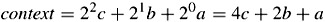For the binary values of a, b and c, the context has a value between 0 and 7. Higher values of the context indicate that a higher probability should be assigned for coding of 1, and a complementary probability, when the value of x is 0.

## A generic interframe video codec

Figure 3.19 shows a generic interframe encoder which is used in all the standard video codecs, such as H.261, H.263, MPEG-1, MPEG-2 and MPEG-4 [20,16,21,22,17]. In the following sections each element of this codec is described in a general sense. The specific aspects of these codecs will be addressed in more detail in the relevant chapters.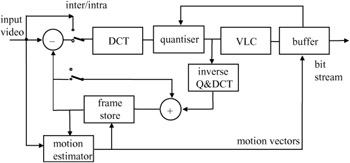Figure 3.19: A generic interframe predictive coder

### Interframe loop

In interframe predictive coding, the difference between pixels in the current frame and their prediction values from the previous frame is coded and transmitted. At the receiver, after decoding the error signal of each pixel, it is added to a similar prediction value to reconstruct the picture. The better the predictor, the smaller the error signal, and hence the transmission bit rate. If the scene is still, a good prediction for the current pixel is the same pixel in the previous frame. However, when there is motion, assuming that movement in the picture is only a shift of object position, then a pixel in the previous frame, displaced by a motion vector, is used.

### Motion estimator

Assigning a motion vector to each pixel is very costly. Instead, a group of pixels are motion compensated, such that the motion vector overhead per pixel can be very small. In standard codecs a block of 16 x 16 pixels, known as a macroblock (MB) (to be differentiated from 8 × 8 DCT blocks), is motion estimated and compensated. It should be noted that motion estimation is only carried out on the luminance parts of the pictures. A scaled version of the same motion vector is used for compensation of chrominance blocks, depending on the picture format.

### Inter intra switch

Every MB is either interframe or intraframe coded, called inter/intra MBs. The decision on the type of MB depends on the coding technique, which will be explained in greater detail in the relevant chapters. For example, in JPEG, all MBs are intraframe coded, as JPEG is mainly used for coding of still pictures.

### DCT

Every MB is divided into 8 × 8 luminance and chrominance pixel blocks. Each block is then transformed via the DCT. There are four luminance blocks in each MB, but the number of chrominance blocks depends on the colour resolutions (image format).

### Quantiser

As mentioned in section 3.2, there are two types of quantiser. One with a dead zone for the AC coefficients and the DC coefficient of inter MB, the other without the dead zone is used for the DC coefficient of intra MB. The range of quantised coefficients can be from -2047 to +2047. With a dead zone quantiser, if the modulus (absolute value) of a coefficient is less than the quantiser step size q it is set to zero, otherwise it is quantised according to eqn 3.6, to generate quantiser indices.

### Variable length coding

The quantiser indices are variable length coded, according to the type of VLC used. Motion vectors, as well as the address of coded macroblocks, are also VLC coded.

### IQ and IDCT

To generate a prediction for interframe coding, the quantised DCT coefficients are first inverse quantised and inverse DCT coded. These are added to their previous picture values (after a frame delay by the frame store), to generate a replica of the decoded picture. The picture is then used as a prediction for coding of the next picture in the sequence.

### Buffer

The bit rate generated by an interframe coder is variable. This is because the bit rate is primarily a function of picture activity (motion of objects and their details). Therefore, to transmit coded video into fixed rate channels (e.g. 2 Mbit/s links), the bit rate has to be regulated. Storing the coded data in a buffer and then emptying the buffer at the channel rate does this. However, if the picture activity is such that the buffer may overflow (violent motion) then a feedback from the buffer to the quantiser can regulate the bit rate. Here, as the buffer occupancy increases, the feedback forces the quantiser step size to be increased to reduce the bit rate. Similarly, if the picture activity is less (coding mainly slow motion parts of frames), then the quantiser step size is reduced to improve the picture quality.

### Decoder

The compressed bit stream, after demultiplexing and variable length decoding (VLD), separates the motion vectors and the DCT coefficients. Motion vectors are used by motion compensation and the DCT coefficients after the inverse quatisation and inverse DCT are converted to error data. They are then added to the motion compensated previous frame, to reconstruct the decoded picture, as shown in Figure 3.20.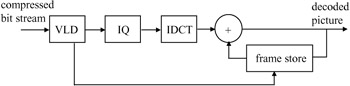Figure 3.20: Block diagram of a decoder

## Constant and variable bit rates

The bit rate generated by the encoder of Figure 3.19 is called a constant bit rate (CBR), requiring a fixed channel bandwidth. An alternative solution is to use a transmission system which can adapt to the variable bit rate (VBR). For VBR coding, the feedback and the smoothing buffer are no longer needed. The quantiser step size in this case is fixed.

In an asynchronous transfer mode (ATM) system , the information is transmitted in the form of fixed length packets or cells and when a cell is full, it is transmitted. This can happen at any time, so that the transmission system has a bit rate capability that matches the encoder. The advantage is realised only when several video channels are multiplexed together. When one channel is generating cells in rapid succession, corresponding to high picture activity, it is probable that the other channels will generate cells at a lower rate. Only rarely will the total bit rate of the multiplex be exceeded and freeze out occur.

## Problems

1.

In the linear quantiser of Figure 3.4, derive the quantisation characteristics in terms of inputs and outputs, for each of the following conditions:

1. th = q = 16
2. th = 0, q = 16.2.

The following eight-bit resolution luminance samples are DPCM encoded with the prediction of previous sample:

• 10, 14, 25, 240, 195, 32

If the quantisation is uniform with th = 0 and q = 8,

1. find the reconstructed samples (assume predictor is initialised to zero)
2. calculate the PSNR of the decoded samples.3.

A three-bit nonuniform quantiser is defined as:

input

output

if |x| ≤ 4

y = ±2

4 < |x| ≤ 10

y = ±6

10 < |x| ≤ 25

y = ±15

else

y = ±50

If the DPCM data of problem 2 are quantised with this quantiser, find the reconstructed samples and the resulting PSNR value.4.

A step function signal with the following eight-bit digitised values:

• 20; 20; 20; 20; 20; 231; 231; 231; 231; 231; 231; 231; 231; 231

is DPCM coded with the nonuniform quantiser of problem 3. Plot the reconstructed samples and identify the positions where slope overload and granular noise occur.5.

Determine the elements of the 8 × 8 orthonormal forward and inverse DCT transformation matrices.6.

Use the DCT matrix of problem 5 to code the following eight pixels:

35; 81; 190; 250; 200; 150; 100; 21

1. find the transform coefficients
2. why is only one AC coefficient significant?
3. reconstruct the pixels without any quantisation; comment on the reconstructed pixel values.7.

The DCT coefficients of problem 6 are linearly quantised with a linear and dead zone quantiser with a step size of th = q = 16. Find the PSNR of the reconstructed pixels.8.

Find the PSNR of the reconstructed pixels, if in problem 7 the following coefficients are retained for quantisation and the remaining coefficients are set to zero:

1. DC coefficient
2. DC and the second AC coefficient.9.

A 2 x 2 block of pixels in the current frame is matched against a similar size block in the previous frame, as shown in Figure 3.21, within a search window of ±2 pixels horizontally and ±1 pixel vertically. Find the best matched motion vector of the block, if the distortion criterion is based on:

1. mean-squared error
2. mean absolute error.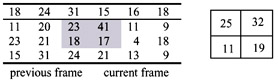Figure 3.21: A block of 2 × 2 pixels in the current frame and its corresponding block in the previous frame shown in the shaded area10.

For a maximum motion speed of six pixels/frame:

1. calculate the number of operations required by the full search method
2. if each block contains 16 × 16 pixels, calculate the number of multiplications and additions, if the cost function was:

1. mean-squared error (MSE)
2. mean absolute error (MAE).11.

Repeat problem 10 for the following fast search methods:

1. TDL
2. TSS
3. CSA
4. OSA12.

Four symbols of a, b, c and d with probabilities p(a) = 0.2, p(b) = 0.45, p(c) = 0.3 and p(d) = 0.05 are Huffman coded. Derive the Huffman codes for these symbols and compare the average bit rate with that of the entropy.13.

In problem 12 a message comprising five symbols cbdad is Huffman coded

1. write down the generated bit stream for this message
2. if there is a single error in the:

1. first bit
2. third bit
3. fifth bit

What is the decoded message in each case?14.

If the intervals of [0.0, 0.2), [0.2, 0.7), [0.7, 0.95) and [0.95, 1) are assigned for arithmetic coding of strings of a, b, c and d respectively, find the lower and upper values of the arithmetic coded string of cbcab.15.

With the interval of strings of a, b, c defined in problem 14, suppose the arithmetic decoder receives 0.83955:

1. decode the first three symbols of the message
2. decode the first five symbols of the message.16.

In arithmetic coding symbols can be decoded using the equation: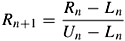where R0 is the received number and [Ln, Un) is the interval of the nth symbol in the stream. Use this equation to decode the symbols in problem 15.17.

Find the binary arithmetic coding of string cbcab of problem 14.18.

Decimal numbers can be represented in binary form by their expansions in powers of 2-1. Derive the first 11 binary digits of the decimal number 0.83955. Compare your results with that of problem 17.19.

The binary digits of the arithmetic coded string cbcab are corrupted at the:

1. first bit
2. third bit
3. fifth bit

Decode the first five symbols of the string in each case.Answers

1.

1. |x| ≤ 16; y = 0 16 < |x| ≤ 32; y = ±24 32 < |x| < 48; y = ±40 etc.
2. |x| ≤ 16; y = ±8 16 < |x| ≤ 32; y = ±24 32 < |x| ≤ 48; y = ±40 etc.

2.

12 16 28 240 196 32 PSNR = 43.4 dB

3.

6 12 27 77 127 77 PSNR = 10.7 dB

4.

15 21 19 21 19 69 119 169 219 234 232 230 232 230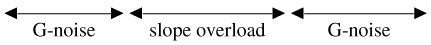5.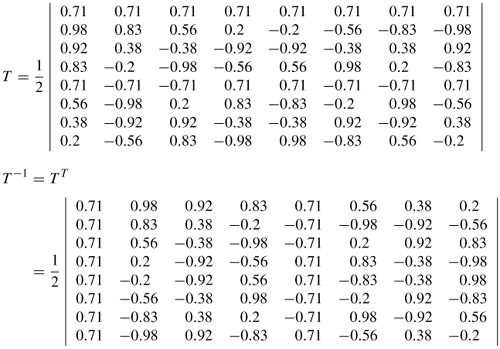6.

1. 364 15 -211 -26 -5 38 -2 -1
2. the basis vector of the second AC coefficient matches the input pixels
3. 35 82 190 250 200 150 101 23. Due to mismatch (approximating the cosine elements) some of the input pixels cannot be reconstructed, e.g. 81/82 and 100/101.

7.

 quantised coefficients: 360 0 -216 -24 0 40 0 0 reconstructed pixels: PSNR = 30 dB 30 70 185 250 204 153 104 27

8.

 reconstructed pixels: PSNR = 10.4 dB 128 128 128 128 128 128 128 128 reconstructed pixels: PSNR = 23.4 dB 28 87 169 227 227 169 87 28

9.

1. mv(-1, -1)
2. mv(-1, -1)

10.

1. 169
1. multiplications = 256 × 169, additions = 511 × 169
2. multiplications = 0, additions = 511 × 169

11.

type

operations

Multiplications

additions

TDL

23

23 × 256

23 × 511

TSS

25

25 × 256

25 × 511

CSA

17

17 × 256

17 × 511

OSA

13

13 × 256

13 × 511

12.

1. = 010,
2. = 1,
3. = 00,
4. = 011, av bits = 1.8, entropy = 1.72

13.

1. cbdad = 001011010011
1. 1st bit in error decoded string = babbad
2. 3rd bit in error, decoded string = ccbbad
3. 5th bit in error, decoded string = cbcbad

14.

lower value = 0.83875, upper value = 0.841875

15.

1. the first three symbols = cbc
2. the first five symbols = cbcab

16.

the same as 15

17.

11010110111

18.

the same as 17

19.

1. first bit in error = 0.01010110111 = 2-2 + 2-4 + 2-6 + 2-7 +2-9 + 2-10 + 2-11 = 0.33935546875 which is decoded to string bbacb
2. similarly, with the third bit in error the decimal number would be 0.96435546875, decoded to dbacb
3. with the fifth bit in error, the decimal number is 0.87060546875 and it is decoded to string cbacb.

## References

1 JAIN, A.K.: 'Fundamentals of digital image processing' (Prentice Hall, 1989)

2 CHEN, W.,SMITH, C., and FRALICK, S.: 'A fast computational algorithm for the discrete cosine transform', IEEE Trans. Commun., 1979, COM-25, pp.1004-1009

3 ISHIGURO, T., and IINUMA, K.: 'Television bandwidth compression transmission by motion-compensated interframe coding', IEEE Commun. Mag., 1982, 10, pp.24–30

4 KAPPAGANTULA, S., and RAO, K.R.: 'Motion compensated predictive coding'. Proceedings of international technical symposium, SPIE, San Diego, CA, August 1983

5 BERGMANN, H.C.: 'Displacement estimation based on the correlation of image segments'. IRE conference on the Electronic image processing, York, U.K., July 1982

6 JAIN, J.R., and JAIN, A.K.: 'Displacement measurement and its application in interframe image coding', IEEE Trans. Commun., 1981, COM-29, pp. 1799–1808

7 SHANABLEH, T., and GHANBARI, M.: 'Heterogeneous video transcoding to lower spatio-temporal resolutions and different encoding formats', IEEE Trans. Multimedia, 2000, 2:2, pp.101–110

8 KOGA, T.,IINUMA, K.,HIRANO, A.,IIJIMA, Y., and ISHIGURO, T.: 'Motion compensated interframe coding for video conferencing'. Proceedings of national Telecommunications conference, New Orleans, LA, November 29-December 3, pp.G5.3.1–G5.3.5

9 CCITT Working Party XV/4: 'Description of reference model 8 (RM8)'. Specialists Group on Coding for Visual Telephony, doc. 525, June 1989

10 SRINIVASAN, R., and RAO, K.R.: 'Predictive coding based on efficient motion estimation'. IEEE International conference on Communications, Amsterdam, May 14–17, 1984, pp.521–526

11 GHANBARI, M.: 'The cross search algorithm for motion estimation', IEEE Trans. Commun., 1990, 38:7, pp.950–953

12 PURI, A.,HANG, H.M., and SCHILLING, D.L.: 'An efficient block-matching algorithm for motion compensated coding'. Proceedings of IEEE ICASSP'87, 1987, pp.25.4.1–25.4.4

13 BIERLING, M.: 'Displacement estimation by hierarchical block matching', Proc. SPIE, Visual Communications and Image Processing, 1988, 1001, pp.942–951

14 LANGDON, G.G.: 'An introduction to arithmetic coding', IBM J. Res. Dev., 1984, 28:2, pp. 135–149

15 PENNEBAKER, W.B., and MITCHELL, J.L.: 'JPEG: still image compression standard' (Van Nostrand Reinhold, New York, 1993)

16 H.263: 'Draft ITU-T Recommendation H.263, video coding for low bit rate communication'. September 1997

17 MPEG-4: 'Testing and evaluation procedures document'. ISO/IEC JTC1/SC29/ WG11, N999, July 1995

18 WITTEN, I.H.,NEAL, R.M., and CLEARY, J.G.: 'Arithmetic coding for data compression', Commun. ACM, 1987, 30:6, pp.520–540

19 GHANBARI, M.: 'Arithmetic coding with limited past history', Electron. Lett., 1991, 27:13, pp.1157–1159

20 H.261: 'ITU-T Recommendation H.261, video codec for audiovisual services at p × 64 kbit/s'. Geneva, 1990

21 MPEG-1: 'Coding of moving pictures and associated audio for digital storage media at up to about 1.5 Mbit/s'. ISO/IEC 1117-2: video, November 1991

22 MPEG-2: 'Generic coding of moving pictures and associated audio information'. ISO/IEC 13818-2 video, draft international standard, November 1994

23 CUTHBURT, A.C., and SAPANEL, J.C.: 'ATM: the broadband telecommunications solution' (IEE publishing, 1993)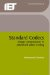Standard Codecs: Image Compression to Advanced Video Coding (IET Telecommunications Series)
ISBN: 0852967101
EAN: 2147483647
Year: 2005
Pages: 148
Authors: M. Ghanbari

Similar book on Amazon

Flylib.com © 2008-2017.
If you may any questions please contact us: flylib@qtcs.net